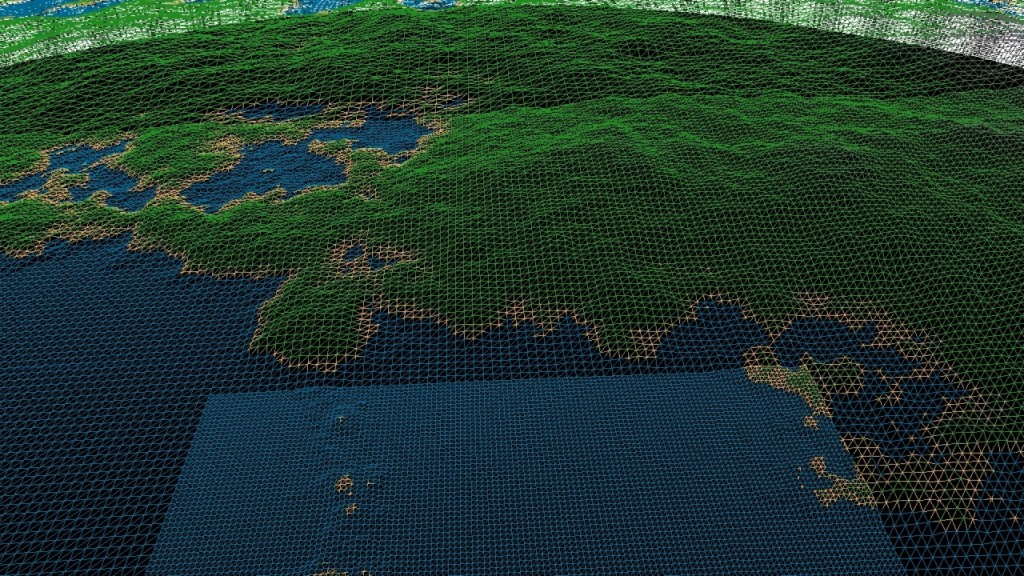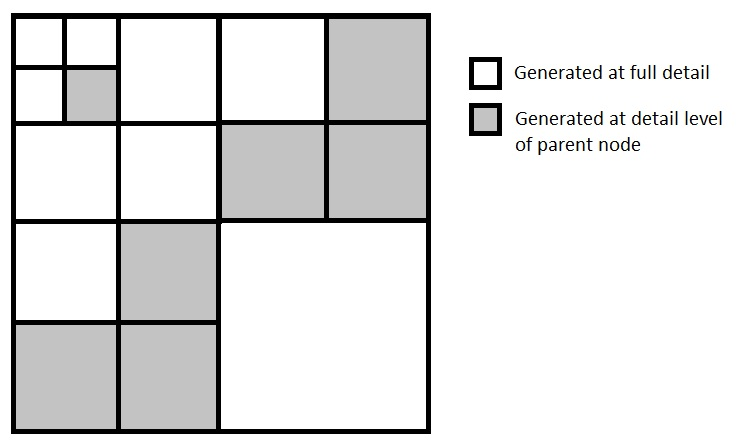# Planetary Scale LOD Terrain GenerationIn the week following my procedural terrain I added a dynamic quadtree-based LOD system in order to be capable of rendering large scale terrain at distances ranging from several kilometers to a few meters.

At this point I ran into the issue that floating point is not accurate enough to accomplish centimeter precision at distances of several hundred thousand meters from 0.0, 0.0, 0.0. I still need to move the height calculations into view space so that they do not lose accuracy.

In order to determine what level of detail I need to use for a given section of terrain I precalculate a list of distances that each LOD level will be within. I do this by starting with the distance that I want to be at before I can see the most detailed tiles. I normally have this base distance at around 50 meters. Then for every level of detail up to the maximum depth that I set, I double this initial value to 100, 200, 400 and so on. Then from the root node I check if the center of the plane is within 50*2^(max level of detail) distance from the camera. If it is then I split the tree into four subsections and check if their center’s distance to the camera is less than 50*2^(max level of detail – 1),  50*2^(max level of detail – 2), … , 50*2^(max level of detail – n) until I have checked the entirety of the tree. This is done every frame and all tiles that are in range get stored in a vector that includes the level of detail that the tile is at, the scale of the tile which is equivalent to 0.5^(node depth), and the offset of the tile which is summed together as the tree is traversed downwards.

I generate a single plane which I draw multiple times with different parameters in order to cover the entire quad tree. This plane has four separate index buffers that specify the indices for four subsections of the mesh that constitute its four quadrants. If a quadrant is within range of the player then it gets split into another node and the indices that constitute that area of the parent node are skipped during drawing.Once the tree has been traversed I draw the mesh for each tile stored in the list of visible tiles. The shaders that draw this take in the offset and scale to transform the plane to fit into its section. After the plane is scaled and offset I project each point onto a sphere using the equation here. Each point is then used as input in a fractional Brownian motion function that uses 3D simplex noise in order to determine the height of the terrain at that point on the unit sphere.

All of the meshes are generated per frame and the program runs at ~200 fps on my GTX 480.